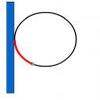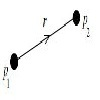#### You may also like### Building up Friction

A series of activities to build up intuition on the mathematics of friction.Can you arrange a set of charged particles so that none of them start to move when released from rest?

# Uniformly Unstable

##### Age 16 to 18 Challenge Level:

Imagine that a real three dimensional object is placed on a smooth flat table. Configurations where the object can exist at rest without moving are called static equilibrium configurations. Some of these static equilibrium configurations are stable: the object will eventually return to the position of equilibrium after a tiny nudge. Some are unstable: the object eventually moves to a new position of equilibrium following a tiny nudge.

Find some solids of essentially uniform density near to you. How many stable and unstable static equilibrium configurations do they have? You could imagine these and then test them out with nudges. Practically speaking many configurations will eventully change with large enough nudges. Perhaps some configurations are more stable than others?

Imagine creating mathematically idealised objects from a material of uniform density. Pick some numbers $S$ and $U$. Can you invent solids with exactly these numbers of stable and unstable equilibrium configurations? For how many different pairs $S$ and $U$ can you devise such a solid? Be sure to describe these idealised solids in a clear, accurate and mathematically precise way.

NOTES AND BACKGROUND

Rather surprisingly, there exists a set of three dimensional shapes of uniform density which have a single stable equilibrium point and a single unstable equilibrium point, called Gombocs. Their existence was conjectured in 1995 and proved in 2006. You can read about their interesting history and relationship with the physical world on Plus Magazine.